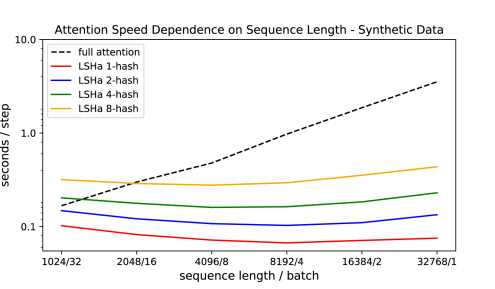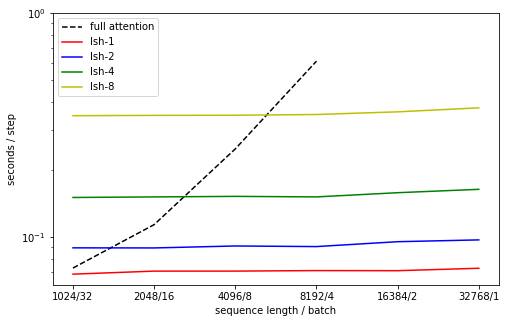from fastai.text.all import *
from reformer_fastai.all import *
from timeit import timeit


We want to test the speed of during evaluation in seconds per step, as reported in the right part of table 5 of the paper: https://arxiv.org/pdf/2001.04451.pdf## get data

Helper method to get data. Assume 1 step of training and 10 of validation.

def get_dataloaders(bs=32, sl=1024, train_steps=1, valid_steps=10, seed=123):
train_sz, valid_sz = bs*train_steps, bs*valid_steps

DeterministicTwinSequence(sl, valid_sz, seed=seed),
bs=bs, shuffle=False, device='cuda')
return dls


## get model

Helper method to get LSHLM method. If n_hashes=0 full attention is used.

def get_lshlm(n_hashes=1, sl=1024, use_lsh=True):
if n_hashes==0: use_lsh=False
max_seq_len=sl,bucket_size=64, n_hashes=n_hashes,
causal=True, use_lsh=use_lsh)


## train

Get a learner that is trained for 1 epoch (just in case).

def get_learner(dls, model, n_epochs=1, lr=1e-3):
loss_func=CrossEntropyLossFlat(ignore_index=-100),
with learn.no_bar():
with learn.no_logging():
learn.fit(n_epochs, lr)
return learn


## time evaluation

'function to get average time per step of validation'
def time_eval(learn,dls, n_rounds=10):
with learn.no_bar():
t = timeit(learn.validate, number=n_rounds)
steps = dls.valid.n / dls.valid.bs
return t / n_rounds / steps


# Loop experiment setup

n_lsh=[0, 1,2,4,8]
sls   =[1024, 2048, 4096, 8192, 16384, 32768]
bss   =[32, 16, 8, 4, 2, 1]
train_steps, valid_steps = 1,10

cols = ['sl', 'bs', 'n-lsh', 'time']
results = []
for sl, bs in zip(sls, bss):
for n_hashes in n_lsh:
if n_hashes==0 and sl>8192:
results.append((sl, bs, n_hashes, np.nan)) # won't fit in memory
else:
dls = get_dataloaders(bs=bs, sl=sl, train_steps=train_steps, valid_steps=valid_steps)
model = get_lshlm(n_hashes=n_hashes, sl=sl)
learn = get_learner(dls, model)
t = time_eval(learn, dls)
del(learn, model, dls)
torch.cuda.empty_cache()
results.append((sl, bs, n_hashes, t))

df = pd.DataFrame(results, columns=cols)

sl bs n-lsh time
0 1024 32 0 0.072765
1 1024 32 1 0.068335
2 1024 32 2 0.089546
3 1024 32 4 0.150253
4 1024 32 8 0.348131
df.to_csv('lsh-timing.csv')

def get_label(nh):
return f'lsh-{nh}' if nh>0 else 'full attention'
def get_linestyle(nh):
return '--' if nh == 0 else '-'

fig, ax = plt.subplots(figsize=(8,5))
for nh, c in zip(n_lsh, ['k','r', 'b', 'g', 'y']):
dat = df.loc[df['n-lsh']==nh]
ax.plot(dat['sl'], dat['time'], color=c, label=get_label(nh), linestyle=get_linestyle(nh))
ax.set_yscale('log')
ax.set_xscale('log', basex=2)

ax.set_xlabel('sequence length / batch')
ax.set_yticks([0.1, 1])
ax.set_xticks(sls)
ax.set_xticklabels(f'{sl}/{bs}' for sl, bs in zip(sls, bss))
ax.legend(loc='upper left')
ax.set_ylabel('seconds / step');We were unable to to do the full sequence length for full attention due to out of memory errors on a single gpu. The results for the smaller sequences are mostly matching, except for lsh-8 which in our experiments turn out slower than in the paper. Also, our full attention seems to be a bit faster. In general results looks offset by a constant. This could be due to method of measurement or architecture choices.